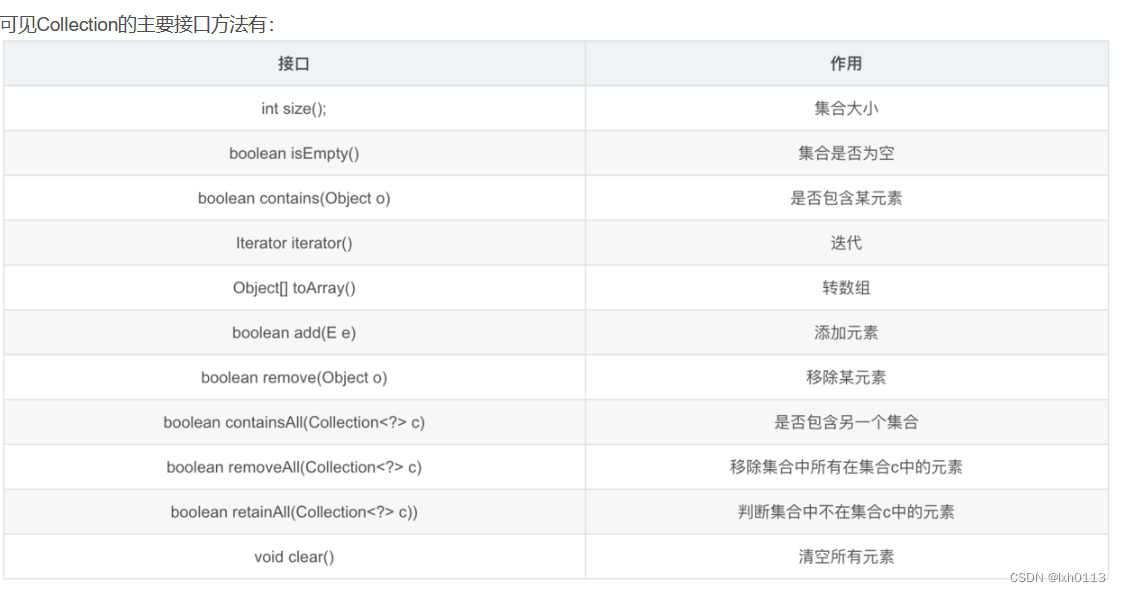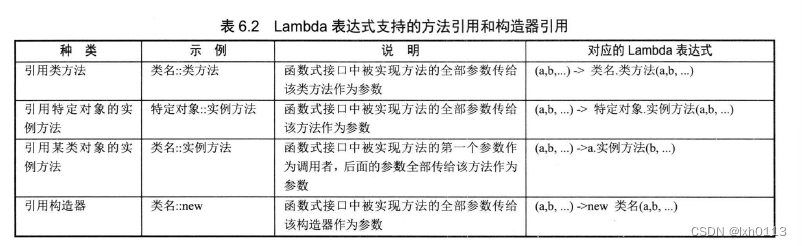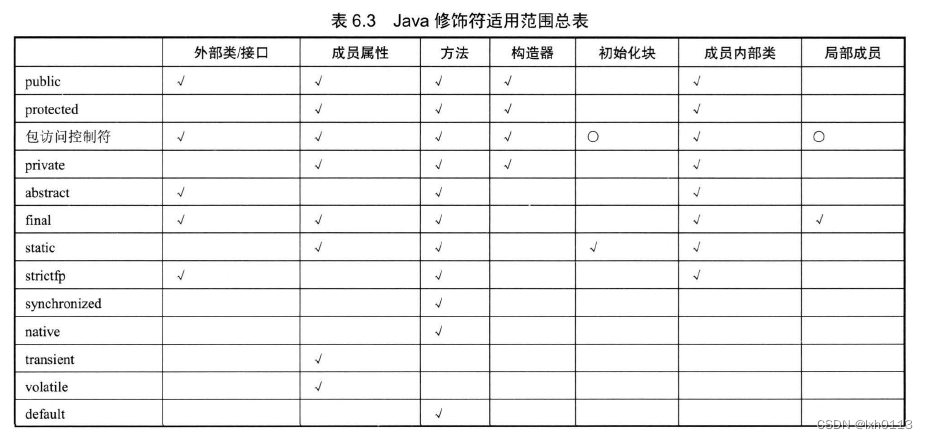# 2023/3/21总结

E - 2xN Grid (atcoder.jp)

1.这一题，就是求出第一行与第二行相对应的相同数字的个数，看数据有多大，我们就知道，这道题目，是不可能穷举的。

2.因此，我们需要 找到规律去写它。此题需要用到前缀和算法。

3.我们需要记录数字和他所在的区间。然后根据区间的位置去判断，该数是否在俩列重合，从而，去相加对应的区间。

``````#include<stdio.h>
#define N 100010
struct node
{
long long x;
long long count;
}a[N];
int main()
{
long long l,n1,n2,i,x,y,j,sum,max;
scanf("%lld%lld%lld",&l,&n1,&n2);
for(i=1;i<=n1;i++)
{
scanf("%lld %lld",&a[i].x,&a[i].count);
a[i].count+=a[i-1].count;
}
for(i=1;i<=n2;i++)
{
scanf("%lld %lld",&a[i].x,&a[i].count);
a[i].count+=a[i-1].count;
}
sum=0;
for(i=1,j=1;i<=n1&&j<=n2;)
{
if(a[i].x==a[j].x)
{
if(a[i].count<a[j].count&&a[j-1].count<a[i].count)
{
if(a[j-1].count>a[i-1].count)
{
max=a[j-1].count;
}
else max=a[i-1].count;

sum+=a[i].count-max;
i++;
}
else if(a[i].count>=a[j].count&&a[j].count>a[i-1].count)
{
if(a[j-1].count>a[i-1].count)
{
max=a[j-1].count;
}
else max=a[i-1].count;

sum+=a[j].count-max;
j++;
}
else if(a[i].count<a[j].count)
{
i++;
}
else if(a[i].count>=a[j].count)
{
j++;
}
}
else if(a[i].count<a[j].count)
{
i++;
}
else if(a[i].count>a[j].count) j++;
else
{
i++,j++;
}
}
printf("%lld\n",sum);
}``````

Problem - B - Codeforces

1.这一题说，只需要比较大小即可，如果它能拿的大，我们可以安排让他先拿完，保证永远比另外一个大

``````#include<stdio.h>
#define N 110
int a[N];
int main()
{
int t,n,i,s1,s2;
scanf("%d",&t);
while(t--)
{
s1=s2=0;
scanf("%d",&n);
for(i=0;i<n;i++)
{
scanf("%d",&a[i]);
if(a[i]%2==0) s1+=a[i];
else s2+=a[i];
}
if(s1>s2) puts("YES");
else puts("NO");
}
return 0;
}``````

Problem - C - Codeforces

1.这道题暴力枚举即可，我们去枚举每一个位置的后一个位置，以及后一个位置每次跳俩次，不相等即可，如果相等就一定是NO，否则是YES

``````#include<stdio.h>
int main()
{
int t,i,j,n;
char s;
scanf("%d",&t);
while(t--)
{
int	flag=1;
scanf("%d",&n);
scanf("%s",s);
for(i=0;i<n&&flag;i++)
{
for(j=i+1;j<n;j+=2)
{
if(s[i]==s[j])
{
flag=0;
break;
}
}
}
if(flag) puts("YES");
else puts("NO");
}
}``````

Problem - D - Codeforces

1.利用前缀和思想，去求出所有的前面的总和，然后减去区间的值，最后加上k*（r-l+1）判断奇数偶数即可

``````#include<stdio.h>
#define N 200010
int a[N];
int b[N];
int main()
{
int t,n,q,i,l,r,k;
scanf("%d",&t);
while(t--)
{
//	sum=0;
b=0;
scanf("%d%d",&n,&q);
for(i=1;i<=n;i++)
{
scanf("%d",&a[i]);
b[i]=a[i]+b[i-1];
}
for(i=0;i<q;i++)
{
scanf("%d%d%d",&l,&r,&k);
//	printf("%d\n",b[n]-(b[r]-b[l-1])+k*(r-l+1));
if((b[n]-(b[r]-b[l-1])+k*(r-l+1))%2) puts("YES");
else puts("NO");
}
}

return 0;
}``````

java知识点

java的控制台输入由system.in()完成。

scanner类用于输入

next()获取字符串类似于c语言里面的scanf(“%s”)，而nextLine()获取字符串很像gets。

FileInputStream和FileOutputStream

InputStream f=new FileInputStream(“工作目录”)

File f=new File(“”);

InputStream in =new FileInputStream(f)

OutputStream f = new FileOutputStream(“”)

File f = new File(“”);

OutputStream fout=new FileOutputSTream(f)

close()

write(int w) throws IOExpception{}

write(byte[] w)new 实现接口() //或者父类构造器(实参列表)

{

//匿名内部类的类体部分

}

Lambda表达式允许使用更简洁的代码来创建一个抽象方法的接口（这种接口被称为函数式接口）

Lambda表达式语法：

(参数) ->(表达式)

(参数)->{ (表达式);}java中枚举类关键字是enum。枚举类不能派生子类。默认是private修饰，也只能是private修饰。

{

……

}